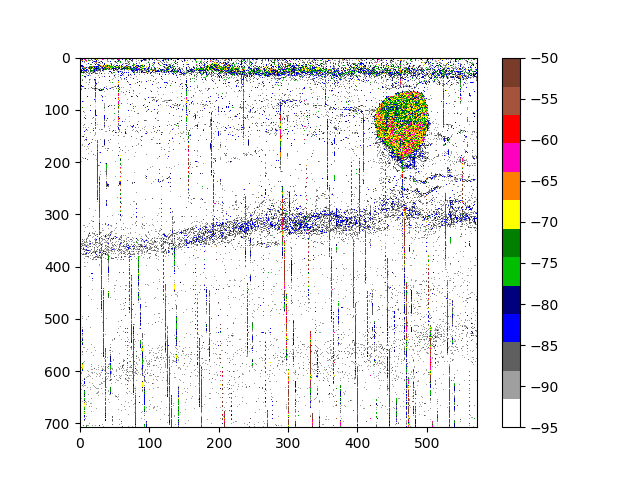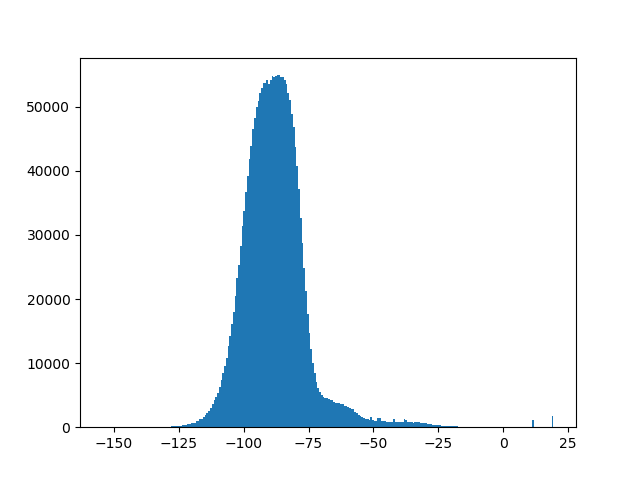0

1

0

1

# EchoJuliaMeta package that loads all the EchoJulia related packages for a "batteries included" hydroacoustic data processing and analysis environment in Julia.

We assume that you have a working Julia 1.0 system, See https://julialang.org/.

## Simple Installation

Switch to package mode by pressing `]`

``````add EchoJulia
``````

## Installation for developers

Clone the repository

``````git clone https://github.com/EchoJulia/EchoJulia.jl.git
``````

Change into the EchoJulia.jl directory

``````cd EchoJulia.jl
``````

Start julia

``````julia
``````

Switch to package mode by pressing `]` and activate, update and precompile:

``````activate .
update
precompile
``````

Quit package mode by pressing `backspace`.

You should now be ready to go.

## Simple Example

Some sample acoustics files are provided for demonstration and testing purposes. `EK60_SAMPLE` is a sample RAW file recorded with a Simrad EK60 scientific echo sounder. `ECS_SAMPLE` is a calibration supplement file containing calibration corrections. The following snippet loads the RAW file, applies calibration and plots a simple echogram:

``````using EchoJulia
data = transform(raw, calibration=cal)
echogram(data["Sv38"], range = maximum(data["r38"]), vmin=-95, vmax=-50, cmap=EK500)
``````## Export to MATLAB

It is often desirable to export the data for further analysis. Note that the MATLAB MAT file format is not a proprietary format, but a specialised HDF5 format.

``````Pkg.add("MAT")

using MAT
matwrite("myfile.mat", data)
``````

NB This seems to be problematic in Julia 1.0 and we are waiting for MAT to be updated.

## Low level API

Although `load` is convenient for exploratory data analysis, we sometimes need access to the underlying EK60 datagrams, for example, to access the survey name or NMEA data.

``````data = collect(datagrams(EK60_SAMPLE))
``````

If performance matters, you might choose to access the data ping by ping:

``````filename = EK60_SAMPLE # or some EK60 RAW file name

ps = pings(filename) # Get the pings
ps38 = [p for p in ps if p.frequency == 38000] # Just 38 kHz pings
Sv38 = Sv(ps38) # Convert to a matrix of volume backscatter
``````

## Histograms

Show a histogram of volume backscatter.

``````eghist(data["Sv38"])
``````## Image processing

EchoJulia uses simple matrices and this allows interoperability with the wider Julia scientific computing tools, especially `Images.jl`.

If you need an echogram image, the following can be useful:

``````using Images
img = imagesc(data["Sv38"])
Images.save("myfile.png", img)
``````

## Dependencies

``````EchoJulia
EchogramImages
EchogramPlots
EchoviewEcs
EchoviewEvr
Filetimes

AliasedSeabed
EchogramUtils
EchogramProcessing

EchogramPyPlot
``````

## Next Steps

EchoJulia is a work in progress with more functionality coming soon. Comments and contributions welcome.

06/05/2018